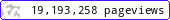•••••MadAsMaths.com :: I.Y.G.B. Practice Papers :: Special & Extension Papers :: M456 Practice Papers

I.Y.G.B. M456 PRACTICE PAPERS

These I.Y.G.B. practice papers mostly follow the Mechanics Edexcel Syllabus introduced for teaching in 2001 and in particular the extinct mechanics modules M4, M5 and M6.

They will offer good practice for able mathematics students who wish to practice topics no longer taught/examined such as relative motion, rotational motion, variable mass systems, polar coordinates to name a few.

• Papers A to R have standard difficulty with later papers usually more difficult.
• Papers S and T are extremely hard.
• Papers U to Z are hard.

All papers have model solutions.

 m456_a.pdfm456_a_solutions.pdfm456_b.pdfm456_b_solutions.pdfm456_c.pdfm456_c_solutions.pdfm456_d.pdfm456_d_solutions.pdfm456_e.pdfm456_e_solutions.pdfm456_f.pdfm456_f_solutions.pdfm456_g.pdfm456_g_solutions.pdfm456_h.pdfm456_h_solutions.pdfm456_i.pdfm456_i_solutions.pdfm456_j.pdfm456_j_solutions.pdfm456_k.pdfm456_k_solutions.pdfm456_l.pdfm456_l_solutions.pdfm456_m.pdfm456_m_solutions.pdfm456_n.pdfm456_n_solutions.pdfm456_o.pdfm456_o_solutions.pdfm456_p.pdfm456_p_solutions.pdfm456_q.pdfm456_q_solutions.pdfm456_r.pdfm456_r_solutions.pdfm456_s.pdfm456_s_solutions.pdfm456_t.pdfm456_t_solutions.pdfm456_u.pdfm456_u_solutions.pdfm456_v.pdfm456_v_solutions.pdfm456_w.pdfm456_w_solutions.pdfm456_x.pdfm456_x_solutions.pdfm456_y.pdfm456_y_solutions.pdfm456_z.pdfm456_z_solutions.pdf